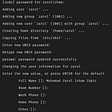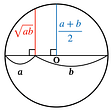# Monoids are Composable List Summarizers

## Examples

• Counts: I can summarize a finite list by counting its elements. If I have the counts of two lists, I can compute the count of their concatenation by adding the two counts together.
• Sums: I can summarize a finite list by its sum. If I have the sum of two lists, I can compute the sum of their concatenation, again by adding.
• Minima and Maxima: I can summarize a finite list by its minimum and maximum elements. (Lists may be empty, but we can define the minimum and maximum of an empty list to be ∞ and -∞, respectively). And again, if I have the minima and maxima of two lists, I can compute the minimum and maximum of their concatenation, by taking the least of the two minima and the greater of the two maxima.
• GCDs: If I’m doing something with number theory, I might summarize a finite list with its greatest common divisor. If I have the greatest common divisors of two lists, I can compute the greatest common divisor of their concatenation, by computing the gcd of the gcds.
• Heads: I can summarize a list by its head (i.e., first element), if it exists! If the list is empty, I will just note that it has no head. Given the heads of two lists, I can tell you the first element of their concatenation: it’s the head of the left-hand list if it exists, and the head of the right-hand list otherwise.
• The Whole List: This one may seem a bit silly, but I’ll reference it later. You can trivially “summarize” a list by just keeping the whole list. Your summarizer here is the identity function! That obviously gives you enough information to know the concatenation, as well, so it’s a perfectly good monoid.

## Counter-examples and how to fix them

• Means: If you’re thinking of ways to summarize a list of numbers, one that probably comes to mind is the mean. The mean is not quite a monoid, because knowing the means of two lists isn’t enough information to compute the mean of their concatenation. Just averaging the averages doesn’t work, unless the lists happen to be the same length!
• Medians: Going down the list of averages, you might also want to know the median of a list. Now things get more dicey, but also more intriguing! Once again, the median itself is not a monoid: knowing the medians of two lists still doesn’t tell you the median of the concatenation.
• First, is that even a monoid? Well, (a) given a list I can always sort it. And (b) If I have the sorted versions of two lists, I can merge them to get the sorted concatenation of those lists. So yes, it’s a monoid.
• Not only is it a monoid, but the monoid structure is helpful. It’s actually easier to merge sorted lists than it is to sort the concatenation of the originals — this is the whole basis of merge sort, after all.
• Finally, this monoid helps with computing a median. Although we don’t save on the size of data, it’s trivial to find the median of a list after sorting it.

## Why is this equivalent to abstract monoids?

• f maps finite lists with elements in E onto S.
• If ⧺ denotes concatenation of finite lists, then f(ab) = f(a) ∗ f(b).
1. The ∗ operation is associative. That is, for any x, y, and z in S, (xy) ∗ z = x ∗ (yz). To show this, we use the fact that f is onto to find lists a, b, and c so that x = f(a), y = f(b), and z = f(c). Then we have this chain of equalities: (xy) ∗ z = (f(a) ∗ f(b)) ∗ f(c) = f(a b) ∗ f(c) = f((a b) c) = f(a (b c)) = f(a) ∗ f(bc) = f(a) ∗ (f(b) ∗ f(c)) = x ∗ (yz).
2. The ∗ operation has an identity. For any x in S, we again use the fact that f is onto to find x with x = f(a). Then if e is the empty list, we have x = f(a) = f(a e) = f(a) ∗ f(e) = x f(e), and similarly on the other side. So f(e) is the identity.
1. That this f is onto. It is, because you can get any x in S by applying f to the one-element list containing x.
2. That f is a monoid homomorphism, so f(ab) = f(a) ∗ f(b). We need only expand the definition of f to get f(a) and f(b) as chains of the monoid operation, and then appeal to the associative property to arrange the parentheses as needed.

## Where next?

• Semigroups are summarizers of nonempty finite lists.
• Commutative monoids are summarizers of bags (aka multisets), where elements can occur more than once, but the order is not significant.
• Magmas (that is, sets with a binary operation but no axioms at all) are summarizers of binary trees.
`type Free :: (Type -> Constraint) -> (Type -> Type)type Free cls a = (forall x. cls x => (a -> x) -> x)type List :: Type -> Typetype List a = Free Monoid atoList :: Foldable f => f a -> List atoList = flip foldMap`

--

--

--

## More from Chris Smith

Software engineer, volunteer K-12 math and computer science teacher, author of the CodeWorld platform, amateur ring theorist, and Haskell enthusiast.

Love podcasts or audiobooks? Learn on the go with our new app.

## Recommended from Medium## JAVA’s Inner Class vs Lambda — The thin line## Bollywood Superstar Deepika Padukone Biography## AuroraFS## Configure Laravel with Deployer## The benefits of managing your Linux Crontab with Git## Chris Smith

Software engineer, volunteer K-12 math and computer science teacher, author of the CodeWorld platform, amateur ring theorist, and Haskell enthusiast.

## The Generalist Argument: On Physics and Programming with types## How to Prove the Law of Sines: a Complete Guide## Visual Proof of Inequality of Arithmetic and Geometric Means (AM–GM Inequality): (a + b)/2 ≥ √ab